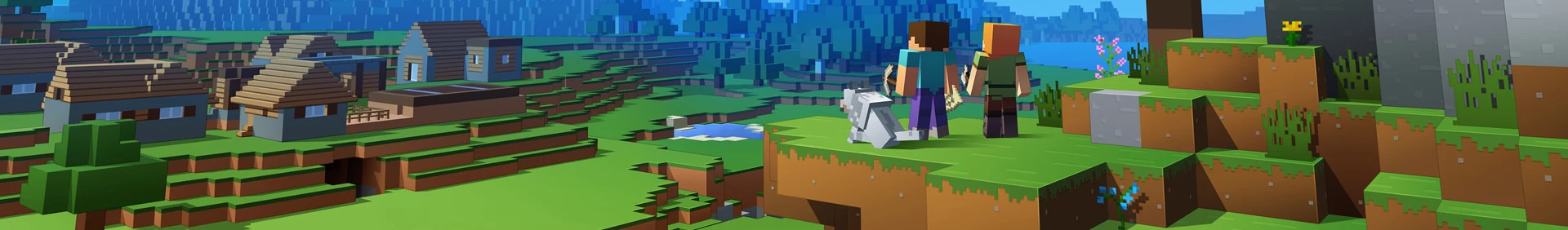# ocxnetdriver

OpenComputers + XNet = :heart:

Allows OpenComputers to utilize XNet networks to transfer items, fluids and energy to connected blocks.

## Known Issues

• This is currently unrestricted, i.e. you can transfer humongous amounts in no time.

## Available Methods

Method Description
getConnectedBlocks() Returns a table with all blocks connected to the XNet network
getSupportedCapabilities(pos[, side]) List all capabilities the given block supports
getItems(pos[, side]) Returns a table with all items in the given inventory
getFluids(pos[, side]) List all fluids in the given tank
getEnergy(pos[, side]) Get capacity and stored energy of the given energy handler
transferItem(sourcePos, sourceSlot, amount, targetPos[, sourceSide[, targetSide]]) Transfer items between two inventories
transferFluid(sourcePos, amount, targetPos[, fluidName][, sourceSide[, targetSide]]) Transfer fluids between two tanks
transferEnergy(sourcePos, amount, targetPos[, sourceSide[, targetSide]]) Transfer energy between two energy handlers
store(sourcePos, sourceSlot, database, entry[, sourceSide]) Store an itemstack from somewhere in the XNet network in a database upgrade

## Synopsis

``````local component = require('component')
local sides = require('sides')
local serialization = require('serialization');

local xnet = component.xnet

-- Dump all found blocks and track all vanilla types in a table
local chests = {}
local tanks = {}
local cells = {}
for i,block in ipairs(xnet.getConnectedBlocks()) do
print(serialization.serialize(block))
if(block.name == "minecraft:chest") then
chests[#chests+1] = block
elseif(block.name == "thermalexpansion:tank") then
tanks[#tanks+1] = block
elseif(block.name == "thermalexpansion:cell") then
cells[#cells+1] = block
end
end
-- {name="thermalexpansion:cell",pos={z=0,y=-1,x=0},side="west",meta=0}
-- {name="opencomputers:case3",pos={z=0,y=-1,x=1},side="north",meta=1}
-- {name="thermalexpansion:tank",pos={z=0,y=0,x=-2},side="down",meta=0,connector="Dynamo"}
-- {name="minecraft:chest",pos={z=1,y=-1,x=-2},side="north",meta=3,connector="Dynamo"}
-- {name="thermalexpansion:dynamo",pos={z=0,y=-1,x=-3},side="east",meta=2,connector="Dynamo"}
-- {name="thermalexpansion:tank",pos={z=0,y=1,x=-1},side="down",meta=0}
-- {name="thermalexpansion:tank",pos={z=0,y=0,x=-2},side="east",meta=0}
-- {name="xnet:controller",pos={z=0,y=0,x=0},side="west",meta=3}

-- Dump the contents of the first chest:
print(serialization.serialize(xnet.getItems(chests.pos)))
-- {
--    {maxSize=64,size=64,hasTag=false,maxDamage=0,damage=0,name="minecraft:stone",label="Stone"},
--    {maxSize=64,size=64,hasTag=false,maxDamage=0,damage=0,name="minecraft:hay_block",label="Hay Bale"},
--    ={maxSize=64,size=1,hasTag=false,maxDamage=0,damage=0,name="xnet:controller",label="Controller"},
--    n=27
-- }

-- Dump the contents of the first tank
print(serialization.serialize(xnet.getFluids(tanks.pos)))
-- {
--    {content={label="Essence of Knowledge",amount=4000,hasTag=false,name="experience"},capacity=20000},
--    n=1
-- }

-- Dump the contents of the first cell
print(serialization.serialize(xnet.getEnergy(cells.pos)))

-- Transfer 5 of whatever is in slot 1 of chest 1 into chest 2
print("Transferred: " .. tostring(xnet.transferItem(chests.pos, 1, 5, chests.pos)))
``````

### Simple energy transfer example

``````local component = require('component')
local sides = require('sides')
local serialization = require('serialization')

local xnet = component.xnet

print(serialization.serialize(xnet.getConnectedBlocks()))
-- {
--    {pos={y=-1,x=0,z=0},name="thermalexpansion:cell",side="west",meta=0},
--    {pos={y=-1,x=1,z=0},name="opencomputers:case3",side="north",meta=1},
--    {pos={y=-1,x=-3,z=0},name="thermalexpansion:dynamo",side="east",meta=2,connector="Dynamo"},
--    {pos={y=0,x=0,z=0},name="xnet:controller",side="west",meta=3},
--    n=4
-- }

-- Find a dynamo and a cell and store their position etc
local energySource = nil
local energyTarget = nil

for i,block in ipairs(xnet.getConnectedBlocks()) do
if(block.name == "thermalexpansion:dynamo") then
energySource = block
elseif(block.name == "thermalexpansion:cell") then
energyTarget = block
end
end

-- Abort if no source could be found
if(energySource == nil) then
print("No dynamo found. Aborting!")
os.exit(1)
end

-- Abort if no target could be found
if(energyTarget == nil) then
print("No cell found. Aborting!")
os.exit(2)
end

-- Transfer 1000RF from source to target using the sides next to the XNet Connector
local transferred, error = xnet.transferEnergy(energySource.pos, 1000, energyTarget.pos, sides[energySource.side], sides[energyTarget.side])
if(transferred ~= nil) then
print(" - Transferred " .. transferred .. "RF to " .. serialization.serialize(energyTarget.pos))
-- - Transferred 1000RF to {y=-1,x=0,z=0}
else
print("Error: " .. error)
end
``````